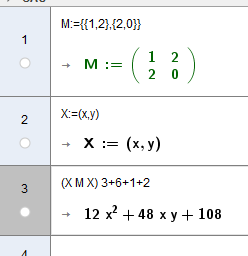hawe shared this problem 1 year ago
In Progress

Line 4-5 ( only variable y evaluated - missing coeffs z)

q_x:=(-y^(2)) - z^(2) - ((2 * y) * z) + (2 * y) - 1

cB:=Coefficients(q_x) ==>cB:={(-1), ((-2) * z) + 2, (-z^(2)) - 1}

AlgebraView ===> {0, (-1), (-1), (-1), 0, 0, (-2), 0, 2, 0} correct

Line 26 (a_0=1 got 5 - have to convert a to list, should be posible to compute Matrix*Vector+Vector?

X M X + a X + a_0 ===> x^(2) + (3 * y^(2)) - z^(2) - ((4 * x) * y) - ((2 * y) * z) + (2 * x) - (2 * y) - 51

Sorry, Coefficients(quadric) only works in the Algebra View (as you've noticed).

For the other problems please say clearly

* which row is wrong

* what you get

* what you'd like to get1

I see,

to Coefficents I have added a additional x^2 to force a correct result.

----

see Line 24 and Line 27

If I add a_0 : X M X + a X + a_0 ===> I got a -5 and not the value of a_0=-1

even

X M X + a X + 1

>x² + 3y² - z² - 4x y - 2y z + 2x - 2y + 5

I am missing a clear statement to convert list <==> vector, vector <==> list

list((1,2,3))={1,2,3}

vector({1,2,3})=(1,2,3)^T

Point({1,2,3})=(1,2,3)

it's pretty horrible to handle homogeneous coordinates in CAS

and Vector <==> Point

v:=Vector( Q)

is possible, but

V:=Point(v)

not....1

V:=Point(v) <-----> V=(0,0,0)+v

v <-----> {x(v),y(v),z(v)}1

Of course you can construct a workaround for almost everything, but in the sense of a clear and readable code it would be desirable to have explicit instructions?

BTW: the functions x(),y(),z() have a low half-life in CAS - and yes, I have workaround for ...1

An other case of matrix vektor additon fails

X=(x,y) , b=(2,0)

{X M X , b X , a_0}

>{x² - 4x y , 2x , -3}

but

X M X + b X + a_0

>x² - 4x y + 4x - 12

see

https://www.geogebra.org/m/tzyzpefa1

* X M X + b X + a_0

* X M X

* b X1

I expect that the addition of

X M X = x² - 4x y

b X = 2x

a_0=-3

will result to

x² - 4x y + 2x -3

and not have the result

x² - 4x y + 4x - 12

see file last posting line 19 ff1

Thanks! For now you'll need to do the calculation in smaller steps I'm afraid1

A workaround is to convert vector b=(2,0) to a list b={2,0}1

big ilogical bug1

he hecho algunas cuentas y en el caso

X M X 3+6+1+2 lo que se calcula realmente es (X M X+9) 12 donde 9=3+6 y 12=3+6+1+2

he probado con otros numeros diferentes y la cuenta se hace de la misma manera

al menos sigue una logica erronea